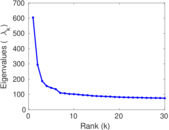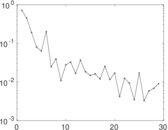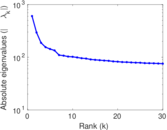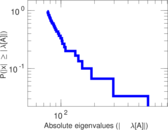These are votes on stories by users of Digg. The network is bipartite, and nodes represent users and stories, and each edge represents one vote. The data covers the period of a month in 2009. The dataset contains multiple edges, when a single user has apparently given multiple votes to a single item.

 Code `DV` Internal name `digg-votes` Name Digg votes Data source http://www.isi.edu/~lerman/downloads/digg2009.html AvailabilityDataset is available for download Consistency checkDataset passed all tests Category Rating network Dataset timestamp 2009 Node meaning User, story Edge meaning Vote Network formatBipartite, undirected Edge typeUnweighted, multiple edges Temporal dataEdges are annotated with timestamps Snapshot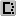Is a snapshot and likely to not contain all data

## Statistics

 Size n = 142,962 Left size n1 = 139,409 Right size n2 = 3,553 Volume m = 3,018,197 Unique edge count m̿ = 3,010,898 Wedge count s = 3,452,748,079 Claw count z = 5,552,161,405,338 Cross count x = 17,710,305,006,153,294 Square count q = 29,370,076,933 4-Tour count T4 = 248,779,120,872 Maximum degree dmax = 24,099 Maximum left degree d1max = 10,526 Maximum right degree d2max = 24,099 Average degree d = 42.223 8 Average left degree d1 = 21.649 9 Average right degree d2 = 849.478 Fill p = 0.006 078 69 Average edge multiplicity m̃ = 1.002 42 Size of LCC N = 142,962 Diameter δ = 4 50-Percentile effective diameter δ0.5 = 3.398 00 90-Percentile effective diameter δ0.9 = 3.879 60 Median distance δM = 4 Mean distance δm = 3.707 10 Gini coefficient G = 0.882 325 Balanced inequality ratio P = 0.126 496 Left balanced inequality ratio P1 = 0.177 512 Right balanced inequality ratio P2 = 0.333 871 Relative edge distribution entropy Her = 0.820 754 Power law exponent γ = 1.572 35 Tail power law exponent γt = 1.771 00 Degree assortativity ρ = −0.179 694 Degree assortativity p-value pρ = 0.000 00 Spectral norm α = 604.628 Algebraic connectivity a = 0.631 175 Controllability C = 135,856 Relative controllability Cr = 0.950 294

## Plots

### Fruchterman–Reingold graph drawing### Degree distribution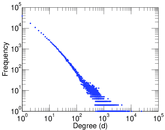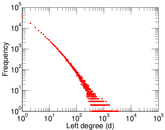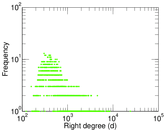### Cumulative degree distribution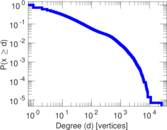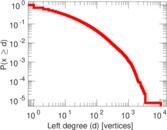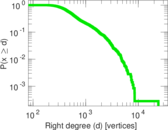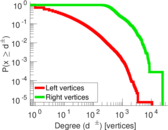### Lorenz curve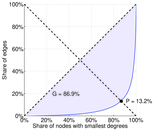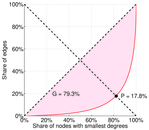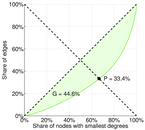### Spectral distribution of the adjacency matrix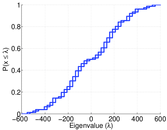### Spectral distribution of the normalized adjacency matrix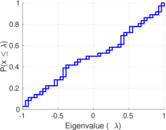### Spectral distribution of the Laplacian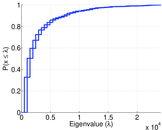### Spectral graph drawing based on the adjacency matrix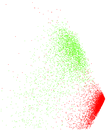### Spectral graph drawing based on the Laplacian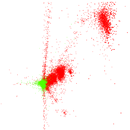### Spectral graph drawing based on the normalized adjacency matrix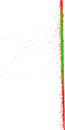### Degree assortativity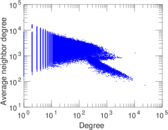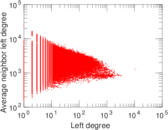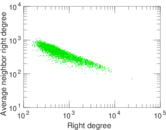### Zipf plot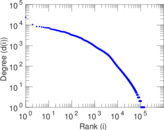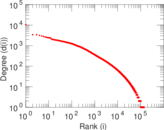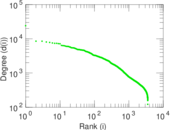### Hop distribution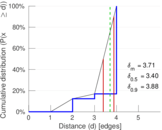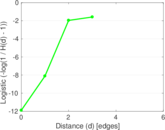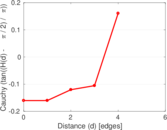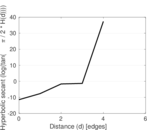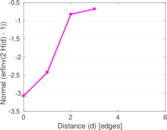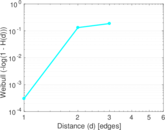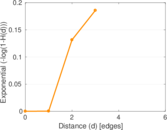### Delaunay graph drawing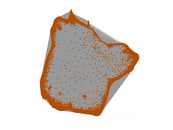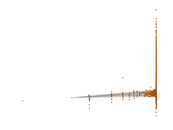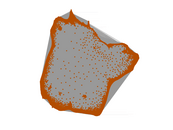### Edge weight/multiplicity distribution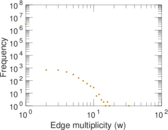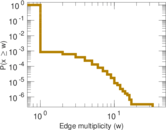### Temporal distribution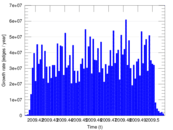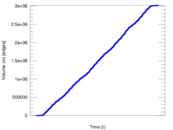### Temporal hop distribution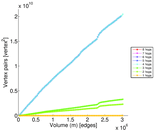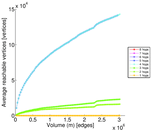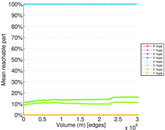### Diameter/density evolution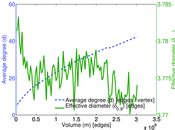### Inter-event distribution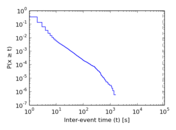### Node-level inter-event distribution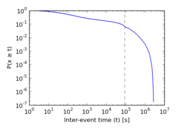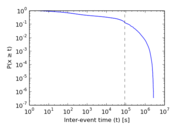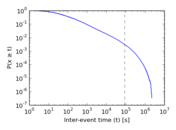### Matrix decompositions plots